Olympiad Test: Paper Cutting

# Olympiad Test: Paper Cutting

Test Description

## 15 Questions MCQ Test Science Olympiad Class 9 | Olympiad Test: Paper Cutting

Olympiad Test: Paper Cutting for Class 9 2022 is part of Science Olympiad Class 9 preparation. The Olympiad Test: Paper Cutting questions and answers have been prepared according to the Class 9 exam syllabus.The Olympiad Test: Paper Cutting MCQs are made for Class 9 2022 Exam. Find important definitions, questions, notes, meanings, examples, exercises, MCQs and online tests for Olympiad Test: Paper Cutting below.
Solutions of Olympiad Test: Paper Cutting questions in English are available as part of our Science Olympiad Class 9 for Class 9 & Olympiad Test: Paper Cutting solutions in Hindi for Science Olympiad Class 9 course. Download more important topics, notes, lectures and mock test series for Class 9 Exam by signing up for free. Attempt Olympiad Test: Paper Cutting | 15 questions in 15 minutes | Mock test for Class 9 preparation | Free important questions MCQ to study Science Olympiad Class 9 for Class 9 Exam | Download free PDF with solutions
 1 Crore+ students have signed up on EduRev. Have you?
Olympiad Test: Paper Cutting - Question 1

### Direction: In each of the following question, a set of three figures X, Y, and Z have been given, showing a sequence in which a paper is folded and finally cut from a particular section. Below these figures a set of answer figures marked (a, b, c, d) showing the design which the paper actually acquires when it is unfolded are given. You have to select the answer figure which most closely resembles the unfolded piece of paper.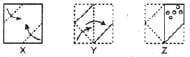Olympiad Test: Paper Cutting - Question 2

### Direction: In each of the following question, a set of three figures X, Y, and Z have been given, showing a sequence in which a paper is folded and finally cut from a particular section. Below these figures a set of answer figures marked (a, b, c, d) showing the design which the paper actually acquires when it is unfolded are given. You have to select the answer figure which most closely resembles the unfolded piece of paper.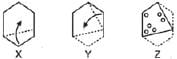Olympiad Test: Paper Cutting - Question 3

### Direction: In each of the following question, a set of three figures X, Y, and Z have been given, showing a sequence in which a paper is folded and finally cut from a particular section. Below these figures a set of answer figures marked (a, b, c, d) showing the design which the paper actually acquires when it is unfolded are given. You have to select the answer figure which most closely resembles the unfolded piece of paper.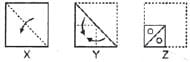Olympiad Test: Paper Cutting - Question 4

Direction: In each of the following question, a set of three figures X, Y, and Z have been given, showing a sequence in which a paper is folded and finally cut from a particular section. Below these figures a set of answer figures marked (a, b, c, d) showing the design which the paper actually acquires when it is unfolded are given. You have to select the answer figure which most closely resembles the unfolded piece of paper.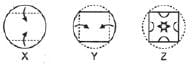Olympiad Test: Paper Cutting - Question 5

Direction: In each of the following question, a set of three figures X, Y, and Z have been given, showing a sequence in which a paper is folded and finally cut from a particular section. Below these figures a set of answer figures marked (a, b, c, d) showing the design which the paper actually acquires when it is unfolded are given. You have to select the answer figure which most closely resembles the unfolded piece of paper.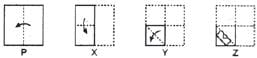Olympiad Test: Paper Cutting - Question 6

Direction: In each of the following question, a set of three figures X, Y, and Z have been given, showing a sequence in which a paper is folded and finally cut from a particular section. Below these figures a set of answer figures marked (a, b, c, d) showing the design which the paper actually acquires when it is unfolded are given. You have to select the answer figure which most closely resembles the unfolded piece of paper.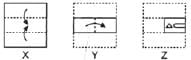Olympiad Test: Paper Cutting - Question 7

Direction: In each of the following question, a set of three figures X, Y, and Z have been given, showing a sequence in which a paper is folded and finally cut from a particular section. Below these figures a set of answer figures marked (a, b, c, d) showing the design which the paper actually acquires when it is unfolded are given. You have to select the answer figure which most closely resembles the unfolded piece of paper.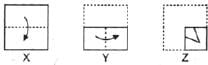Olympiad Test: Paper Cutting - Question 8

Direction: In each of the following question, a set of three figures X, Y, and Z have been given, showing a sequence in which a paper is folded and finally cut from a particular section. Below these figures a set of answer figures marked (a, b, c, d) showing the design which the paper actually acquires when it is unfolded are given. You have to select the answer figure which most closely resembles the unfolded piece of paper.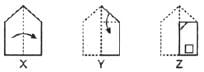Olympiad Test: Paper Cutting - Question 9

Direction: In each of the following question, a set of three figures X, Y, and Z have been given, showing a sequence in which a paper is folded and finally cut from a particular section. Below these figures a set of answer figures marked (a, b, c, d) showing the design which the paper actually acquires when it is unfolded are given. You have to select the answer figure which most closely resembles the unfolded piece of paper.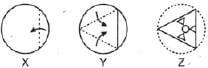Olympiad Test: Paper Cutting - Question 10

Direction: In each of the following question, a set of three figures X, Y, and Z have been given, showing a sequence in which a paper is folded and finally cut from a particular section. Below these figures a set of answer figures marked (a, b, c, d) showing the design which the paper actually acquires when it is unfolded are given. You have to select the answer figure which most closely resembles the unfolded piece of paper.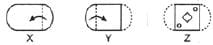Olympiad Test: Paper Cutting - Question 11

Direction: In each of the following question, a set of three figures X, Y, and Z have been given, showing a sequence in which a paper is folded and finally cut from a particular section. Below these figures a set of answer figures marked (a, b, c, d) showing the design which the paper actually acquires when it is unfolded are given. You have to select the answer figure which most closely resembles the unfolded piece of paper.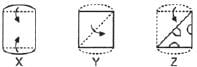Olympiad Test: Paper Cutting - Question 12

Direction: In each of the following question, a set of three figures X, Y, and Z have been given, showing a sequence in which a paper is folded and finally cut from a particular section. Below these figures a set of answer figures marked (a, b, c, d) showing the design which the paper actually acquires when it is unfolded are given. You have to select the answer figure which most closely resembles the unfolded piece of paper.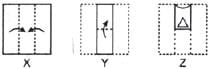Olympiad Test: Paper Cutting - Question 13

Direction: In each of the following question, a set of three figures X, Y, and Z have been given, showing a sequence in which a paper is folded and finally cut from a particular section. Below these figures a set of answer figures marked (a, b, c, d) showing the design which the paper actually acquires when it is unfolded are given. You have to select the answer figure which most closely resembles the unfolded piece of paper.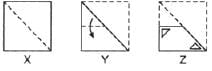Olympiad Test: Paper Cutting - Question 14

Direction: In each of the following question, a set of three figures X, Y, and Z have been given, showing a sequence in which a paper is folded and finally cut from a particular section. Below these figures a set of answer figures marked (a, b, c, d) showing the design which the paper actually acquires when it is unfolded are given. You have to select the answer figure which most closely resembles the unfolded piece of paper.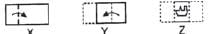Olympiad Test: Paper Cutting - Question 15

Direction: In each of the following question, a set of three figures X, Y, and Z have been given, showing a sequence in which a paper is folded and finally cut from a particular section. Below these figures a set of answer figures marked (a, b, c, d) showing the design which the paper actually acquires when it is unfolded are given. You have to select the answer figure which most closely resembles the unfolded piece of paper.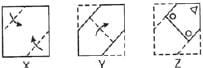## Science Olympiad Class 9

17 videos|6 docs|55 tests
 Use Code STAYHOME200 and get INR 200 additional OFF Use Coupon Code
Information about Olympiad Test: Paper Cutting Page
In this test you can find the Exam questions for Olympiad Test: Paper Cutting solved & explained in the simplest way possible. Besides giving Questions and answers for Olympiad Test: Paper Cutting , EduRev gives you an ample number of Online tests for practice

## Science Olympiad Class 9

17 videos|6 docs|55 tests